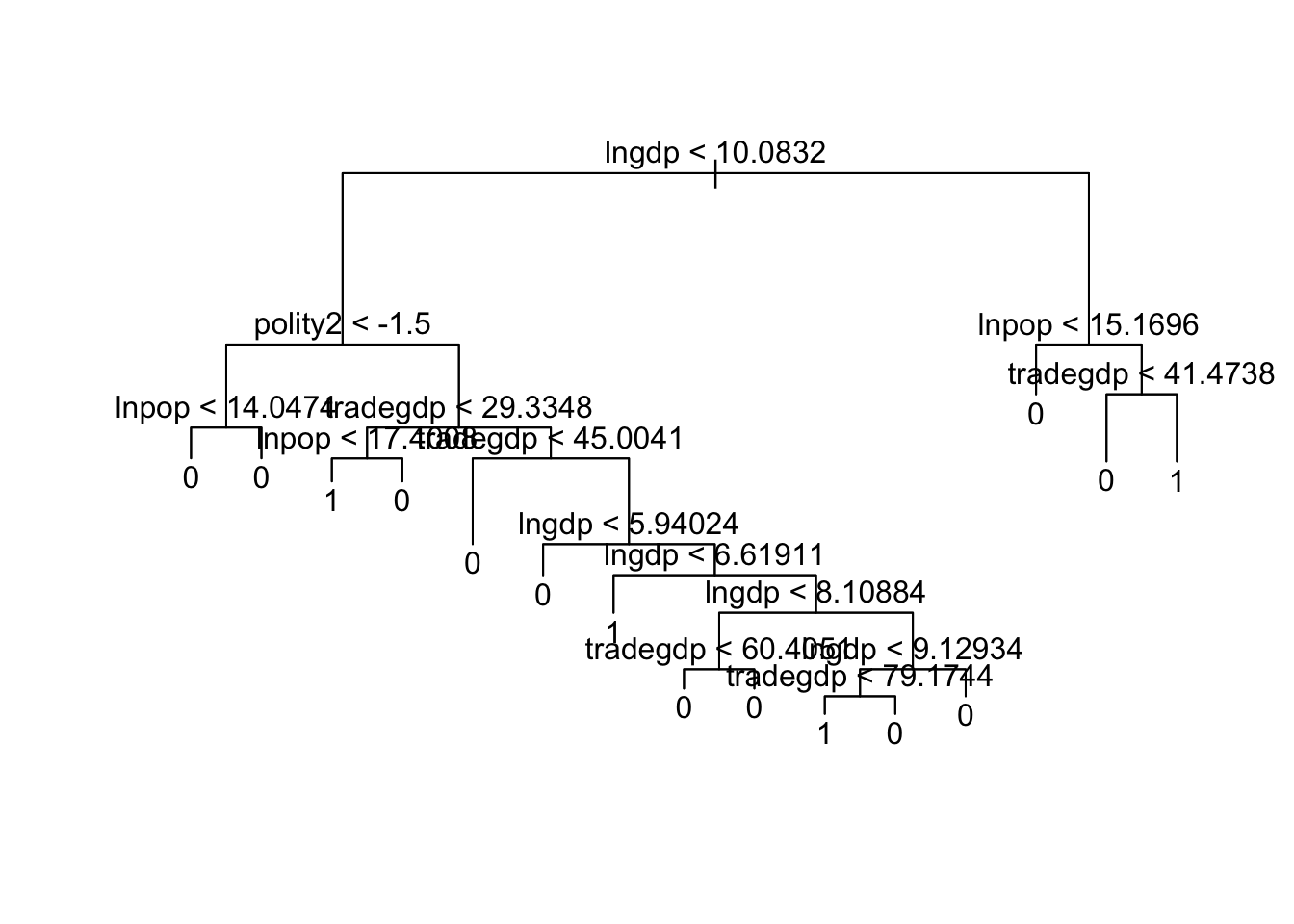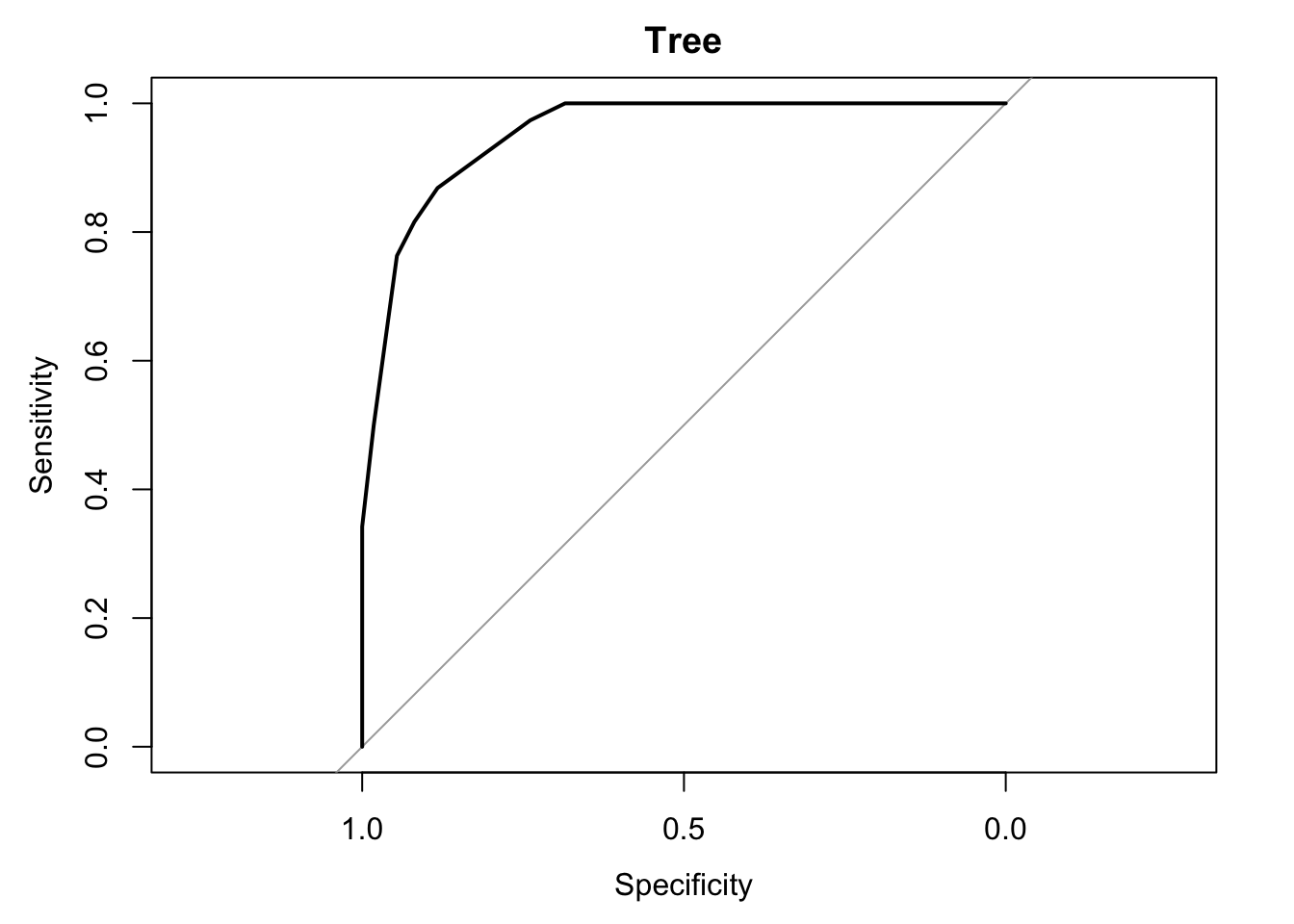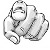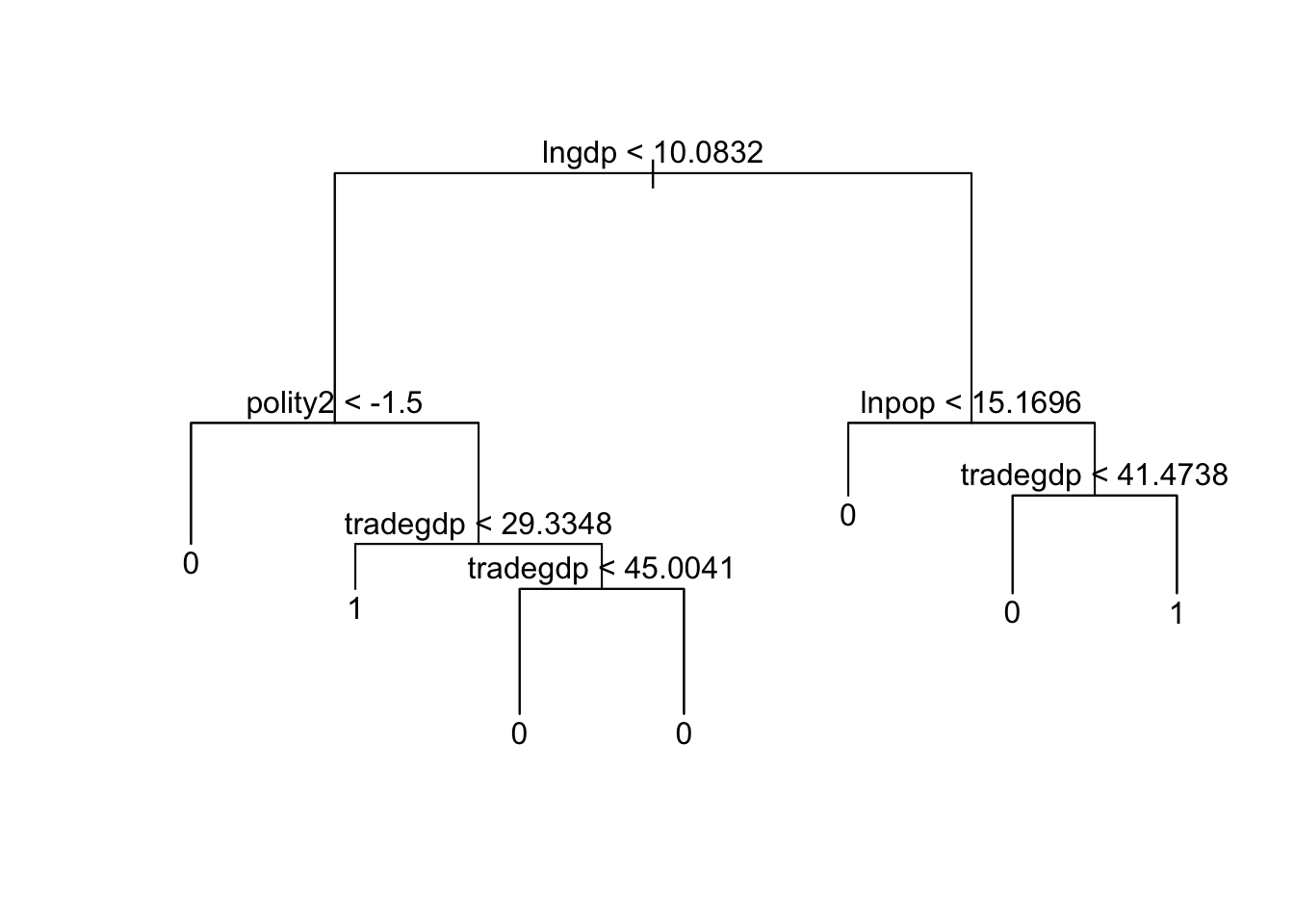Opening data

suppressMessages({
library(rio)
library(stargazer)
library(ggplot2)
library(MASS)
library(knitr)
library(tree)
library(randomForest)
library(pROC)
})

ross <- import("ReplicationdataRossVoeten.dta")
icc <- import("http://www.joselkink.net/files/data/icc.dta")

ross\$iccSigned <- 0
ross\$iccRatified <- 0
for (i in 1:dim(icc)) {
ross\$iccSigned[ross\$ccode == icc\$ccode[i] & ross\$Year >= icc\$signed[i]] <- 1
ross\$iccRatified[ross\$ccode == icc\$ccode[i] & ross\$Year >= icc\$ratified[i]] <- 1
}

summary(ross\$Year)
##    Min. 1st Qu.  Median    Mean 3rd Qu.    Max.
##    1960    1973    1987    1987    2001    2014
length(unique(ross\$countryname))
##  171
ross2001 <- subset(ross, Year == 2001)

So there are 171 countries over a time period from 1960 to 2014 - although of course not all variables will be available for all countries and all years.

The above code also downloads a small data file on membership to the International Criminal Court, a binary variable reflecting whether a country signed the treaty and a binary variable whether the country ratified it. For 2001 the distribution is as follows:

tbl <- table(ross2001\$iccSigned, ross2001\$iccRatified)
colnames(tbl) <- c("Not ratified", "Ratified")
rownames(tbl) <- c("Not signed", "Signed")
Not ratified Ratified Sum
Not signed 88 1 89
Signed 60 45 105
Sum 148 46 194
Not ratified Ratified Sum
Not signed 98 1 99
Signed 57 42 99

So 42% of the signed treaties are subsequently ratified.

To avoid complications that are common in panel data, for the remainder of this lab we just use data from 2001.

We run the linear regression from last class just to get the design matrix:

m1 <- lm(iccRatified ~ polity2 + logoil + lngdp + tradegdp + lnpop, data = ross2001)
designMatrix <- m1\$model

Tree classification

We continue with the same model we used last class for classification, explaining whether or not countries ratified the ICC treaty.

t <- tree(as.factor(iccRatified) ~ polity2 + logoil + lngdp + tradegdp + lnpop, data = ross2001)
plot(t)
text(t)plot(roc(designMatrix\$iccRatified, predict(t)[,2]), main = "Tree")Compare this to the ROC curves from the previous lab. How does it compare?

This tree has a lot of branches, so it is difficult to interpret the output. It would probably be worthwhile to prune the tree to, say, 6 branches:

t6 <- prune.tree(t, best = 6)
plot(t6)
text(t6)Interpret the plot.

Random forests

Instead of using just one tree, we can use a forest of trees. This increases predictive quality, but at the cost of interpretativeness.

f <- randomForest(as.factor(iccRatified) ~ polity2 + logoil + lngdp + tradegdp + lnpop, data = designMatrix)

importance(f)
##          MeanDecreaseGini
## polity2         10.685924
## logoil           6.678683
## lngdp           17.017069